## Line Drawing Algorithms-

In computer graphics, popular algorithms used to generate lines are-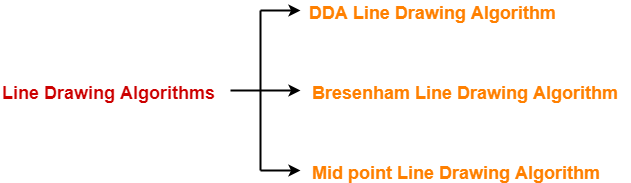1. Digital Differential Analyzer (DDA) Line Drawing Algorithm
2. Bresenham Line Drawing Algorithm
3. Mid Point Line Drawing Algorithm

## DDA Algorithm-

DDA Algorithm is the simplest line drawing algorithm.

 Given the starting and ending coordinates of a line,DDA Algorithm attempts to generate the points between the starting and ending coordinates.

### Procedure-

Given-

• Starting coordinates = (X0, Y0)
• Ending coordinates = (Xn, Yn)

The points generation using DDA Algorithm involves the following steps-

### Step-01:

Calculate ΔX, ΔY and M from the given input.

These parameters are calculated as-

• ΔX = Xn – X0
• ΔY =Yn – Y0
• M = ΔY / ΔX

### Step-02:

Find the number of steps or points in between the starting and ending coordinates.

if (absolute (ΔX) > absolute (ΔY))

Steps = absolute (ΔX);

else

Steps = absolute (ΔY);

### Step-03:

Suppose the current point is (Xp, Yp) and the next point is (Xp+1, Yp+1).

Find the next point by following the below three cases-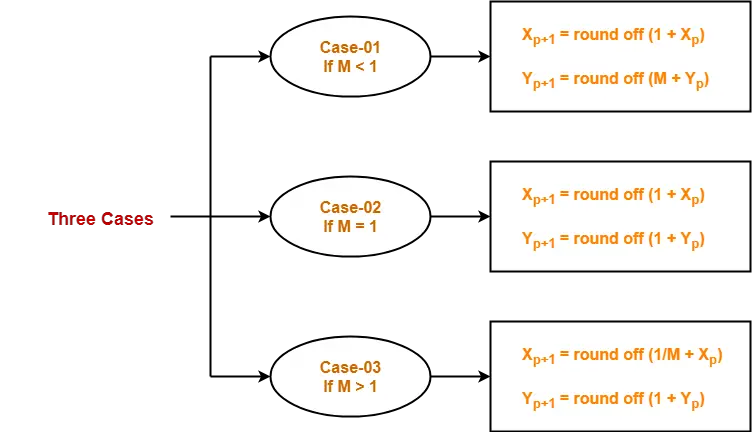### Step-04:

Keep repeating Step-03 until the end point is reached or the number of generated new points (including the starting and ending points) equals to the steps count.

## Problem-01:

Calculate the points between the starting point (5, 6) and ending point (8, 12).

## Solution-

Given-

• Starting coordinates = (X0, Y0) = (5, 6)
• Ending coordinates = (Xn, Yn) = (8, 12)

### Step-01:

Calculate ΔX, ΔY and M from the given input.

• ΔX = Xn – X0 = 8 – 5 = 3
• ΔY =Yn – Y0 = 12 – 6 = 6
• M = ΔY / ΔX = 6 / 3 = 2

### Step-02:

Calculate the number of steps.

As |ΔX| < |ΔY| = 3 < 6, so number of steps = ΔY = 6

### Step-03:

As M > 1, so case-03 is satisfied.

Now, Step-03 is executed until Step-04 is satisfied.

 Xp Yp Xp+1 Yp+1 Round off (Xp+1, Yp+1) 5 6 5.5 7 (6, 7) 6 8 (6, 8) 6.5 9 (7, 9) 7 10 (7, 10) 7.5 11 (8, 11) 8 12 (8, 12)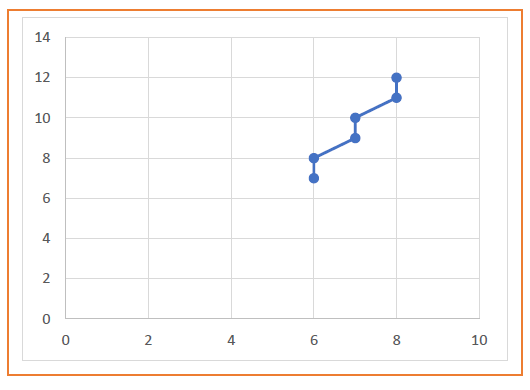## Problem-02:

Calculate the points between the starting point (5, 6) and ending point (13, 10).

## Solution-

Given-

• Starting coordinates = (X0, Y0) = (5, 6)
• Ending coordinates = (Xn, Yn) = (13, 10)

### Step-01:

Calculate ΔX, ΔY and M from the given input.

• ΔX = Xn – X0 = 13 – 5 = 8
• ΔY =Yn – Y0 = 10 – 6 = 4
• M = ΔY / ΔX = 4 / 8 = 0.50

### Step-02:

Calculate the number of steps.

As |ΔX| > |ΔY| = 8 > 4, so number of steps = ΔX = 8

### Step-03:

As M < 1, so case-01 is satisfied.

Now, Step-03 is executed until Step-04 is satisfied.

 Xp Yp Xp+1 Yp+1 Round off (Xp+1, Yp+1) 5 6 6 6.5 (6, 7) 7 7 (7, 7) 8 7.5 (8, 8) 9 8 (9, 8) 10 8.5 (10, 9) 11 9 (11, 9) 12 9.5 (12, 10) 13 10 (13, 10)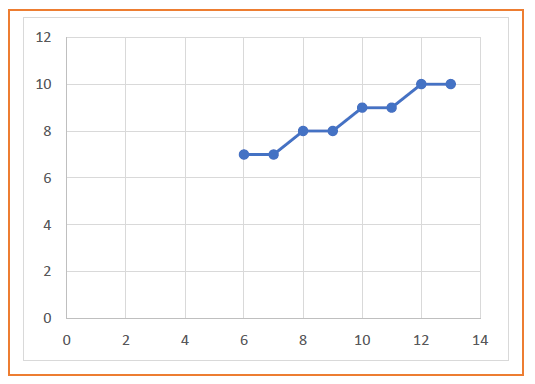## Problem-03:

Calculate the points between the starting point (1, 7) and ending point (11, 17).

## Solution-

Given-

• Starting coordinates = (X0, Y0) = (1, 7)
• Ending coordinates = (Xn, Yn) = (11, 17)

### Step-01:

Calculate ΔX, ΔY and M from the given input.

• ΔX = Xn – X0 = 11 – 1 = 10
• ΔY =Yn – Y0 = 17 – 7 = 10
• M = ΔY / ΔX = 10 / 10 = 1

### Step-02:

Calculate the number of steps.

As |ΔX| = |ΔY| = 10 = 10, so number of steps = ΔX = ΔY = 10

### Step-03:

As M = 1, so case-02 is satisfied.

Now, Step-03 is executed until Step-04 is satisfied.

 Xp Yp Xp+1 Yp+1 Round off (Xp+1, Yp+1) 1 7 2 8 (2, 8) 3 9 (3, 9) 4 10 (4, 10) 5 11 (5, 11) 6 12 (6, 12) 7 13 (7, 13) 8 14 (8, 14) 9 15 (9, 15) 10 16 (10, 16) 11 17 (11, 17)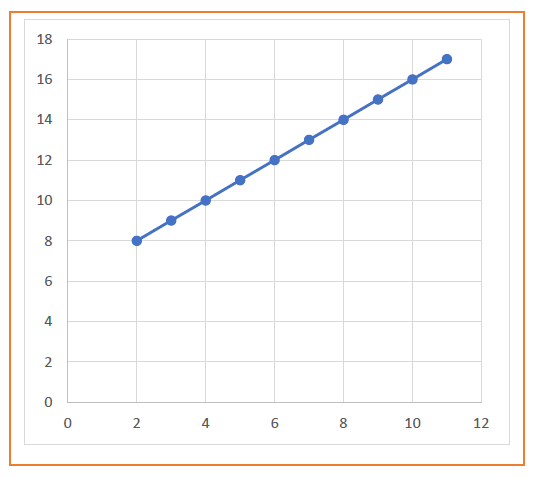The advantages of DDA Algorithm are-

• It is a simple algorithm.
• It is easy to implement.
• It avoids using the multiplication operation which is costly in terms of time complexity.

The disadvantages of DDA Algorithm are-

• There is an extra overhead of using round off( ) function.
• Using round off( ) function increases time complexity of the algorithm.
• Resulted lines are not smooth because of round off( ) function.
• The points generated by this algorithm are not accurate.

To gain better understanding about DDA Algorithm,

Watch this Video Lecture

Next Article- Bresenham Line Drawing Algorithm

Get more notes and other study material of Computer Graphics.

Watch video lectures by visiting our YouTube channel LearnVidFun.